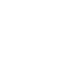# Mar 14 ACT & SAT Question of the Day

If you are connecting to my blog using an email you received from me, do not click the link to sat.collegeboard.org below. Use the link to my website that is farther down on the email.

http://sat.collegeboard.org/practice/sat-question-of-the-day?src=R&questionId=20130314 (This link takes you to today’s question. If you use my archive, you will see the question related to my SAT explanation for that date.)

The answer is C.  Gosh, you sure could do the question following the explanation provided by the SAT folks but why would you do that?  It takes a lot of time and you could make a simple math mistake along the way.  There is certainly an easier way to do it

Just use a couple of the Wizard’s strategies.  First, since it is a circle question, the key to answering it will be the radii of the circles.  Just looking at the circles (drawn to scale) and then reading the information, you know the radius of the small circle is half that of the larger circle.  (You can see the diameter of the small circle is the same as the radius of the large circle.  That makes the radius of the small one half as big as the radius of the big one.)  That means the ratio between the two radii is 1:2.

Second, just plug in the ratios of the radii into the formula for the area of a circle.  1 squared is 1 pi and 2 squared is 4 pi.  That shows you the big circle is 4 times as big.  48/4 is 12, answer C.

There’s even an easier way to figure it out.  Just plug the increase in size from the small to the large circle into the area of a circle formula.  It is twice as big; so, plug 2 for r into the formula.  You have to square 2 and so you know it will be four times as big.  Again, divide by 4 and you get 12, answer C.

This process always works.  Just plug the relative size into the equation for whatever figure you are given.  For example, what if you have two cubes and the length of the sides are 3 and 6?  The large cube’s side is two times as big.  Plug 2 into the equation for the volume of a cube.  2 cubed is eight.  The big cube’s volume is 8 times that of the small cube.  Practice this technique and build your confidence.  Your confidence will increase your score.

Let’s move on to see what the ACT folks have for us this morning.

http://www.act.org/qotd/ (The ACT staff does not put a date on their questions so if you click on an archived blog, you’ll get today’s question and the old explanation. Sorry. The SAT staff has dated their questions; so, the archive is helpful. The ACT folks simply don’t do that.)

The answer is A.  There’s nothing wrong with the sentence.  When there is “NO CHANGE” to the underlined part of the sentence on both the ACT and SAT, the question can be among the hardest ones to get correct.  That is because we are all expecting a mistake and make one up when there isn’t one!  There will be some quesitons on each test that you don’t have to fix or identify a mistake.  On the SAT, they are frequently towards the end of each grammar format since questions are organized from easy to hard on the SAT.  (The exception is the SAT “fix the passage” questions which are organized chronologically in sync with the passage.)  However, on the ACT, they can be anywhere since there is no organization based on difficulty.

I hope my LNHS students are watching the ACT DVD for Monday’s class.  Have a great day.

The Wizard